Home MonkeyNotes Printable Notes Digital Library Study Guides Study Smart Parents Tips College Planning Test Prep Fun Zone Help / FAQ How to Cite New Title Request

 Chapter 2 :Elements (1) Write a short note on Elements. (2) Explain the diagonal relationship in the periodic table. (3) Give the atomic weight and the atomic number of the following elements from the periodic table.      Sodium, Iron, Zinc, Tin, Sulfur, Lead (4) Write a short note on the characteristics of      s-block, p-block and d-block elements. (5) Write a short note on the periodic table. Chapter 3 : Atomic Structure (1) What possible values will other quantum numbers assume when the principle quantum number is 3 ? [ Ans. 0, 1, 2 ] (2) Write notes on : principle quantum number orbital quantum number atomic number and mass number (3) Write the electronic configuration of the following atoms Beryllium Carbon Neon Magnesium (4) Arrange the following in the increasing order of energy.       3p      4p      4s      3d      2p      3s      2s Chapter 4 : Chemical Bondage (1) Using the electronegativity chart interpret the bonding in CS2, CaO, PBr3, NaH, AlCl3 (2) Draw a Lewis diagram for electronic configuration of PCl3, PCl5, Methyl acetylene. (3) State the octet rule and its inadequecies. (4) Define ionic and covalent bond. (5) Describe hydrogen bonding. (6) Compare the bond strength in following compounds : i) NaCl and NaI ii) BaO and BaCl2 iii) LiF and CsF (7) Which member of the following pair will have the larger size: i) Strontium and Rubidium ii) Magnesium and Phosphorous iii) Boron and Oxygen iv) Potassium and Cesium v) Fluorine and Iodine. Chapter 5 : States of Matter (1) Explain the different states of matter and their properties. (2) What is a phase diagram? Explain the phase diagram of water. (3) Define triple points and critical points. (4) Explain why camphor sublimes and paraffin melts by warming at normal pressure. (5) What is heat capacity ? (6) Calculate the amount of heat required to heat 585 gms of MgO from 420C to 540C. (7) Calculate the amount of heat required to heat 10 moles of CaCO3 from 30.50 C to 350C. Chapter 6 : Gases (1) What is the pressure of 11 gm of CO2 if the volume is 15.6 dm3 at 2980K? (mol. weight of CO2 = 44, R = 8.314 J K-1 mol-1) [ Ans. 39.7 KPa ] (2) A balloon contains a certain amount hydrogen gas at atmospheric pressure and 300 K. If the volume of the balloon is 1.6 dm3, how many moles of the gas were present in the balloon? (R = 8.314 J K-1mol-1). [ Ans. 0.065 mol ] (3) What is the final volume if 5 dm3 of a gas at 202.6 KPa pressure is allowed to reach a pressure of 75.98 KPa ? The temperature and mass are held constant. [ Ans.13.33 dm3] (4) What is the final temperature of 10 dm3 of argon at 288 K if it is compressed to 3.5 dm3 ? [Ans. 100.80K ] (5) How many molecules are present in 128 gm of SO2 (mol.weight of SO2 = 64)? [ Ans. 12.046 ´ 1023 ] (6) What are the properties of gases? (7) What is ideal gas equation? Derive its formula. (8) State all five gas laws. (9)  Describe Avogadros number. Chapter 7 : Redox Reactions (1) Write the oxidation number of : (a) S in H2S, H2SO3 , S2O3-2, SO3 (b) Mn in KMnO4, MnO4-, MnO2 , Mn2O3 (c) Cl in KClO3, HCl (2) Define oxidation number of an element. (3) Explain the term redox reaction with examples. (4) Balance following reactions : (a) Zn + NO3- + H+ ® Zn2+ + NH4+ + H2O (b) ClO3- + SO2 ® ClO2 + HSO4- (c) P + HNO3 ® H3PO4 + NO (d) HIO3 + H2SO3 ® I2 + H2SO4 + H2O Chapter 8 : Solutions 1) Define or explain following terms. i) Solvent and Solute ii) Solubility of a substance iii) Saturated and unsaturated solutions iv) Five ways of expressing concentration of a solution v) Elevation of boiling point vi) Depression of freezing point vii) Heat of solution. 2) What is the boiling point of a solution prepared by dissolving 0.300 g of a compound (mol.wt : 128) in 30 gms of carbon tetrachloride ? [ Ans. 77.190C ] 3) What will be the molecular weight of a solute if it elevates the boiling point of 75 gms of benzene by 0.50C and depresses the freezing point by 1.060C when 2.4 g of solute is dissolved in it? [ Ans. 154 ] Chapter 9 : Electrochemistry (1) What is electrolysis ? (2) State Faradays first and second law of electrolysis and explain with examples. (3) Explain the process of electrolysis in Sodium chloride. (4) What is an electrochemical cell and the Daniell cell ? (5) Differentiate between electrolytic cell and electrochemical cell. (6) What is reference electrode ? (7) Define EMF series, electrochemical equivalent and standard oxidation potential. (8) A current of 0.5 ampere was passed through a solution of copper sulphate for 60 minutes. Calculate the weight of copper deposited in the cathode ( ECE of Cu = 0.0003294 gms ). [ Ans. 0.5929 gms ] (9) Standard reduction potentials of Cu and Ag are 0.34 and 0.8 Respectively. What will be the EMF of the cell ? [ Ans. 0.46 V] (10) How much current will be required to liberate 10 g of iodine from potassium iodide solution in 2 hours ? (Eq. wt. of I2 = 127.0) [ Ans. 1.06 amp ] (11) 0.079 f of a metal was deposited by a current of 0.2 ampere in 20 minutes. What is its electrochemical equivalent ? [ Ans. 0.00033 ] Chapter 10 : Elements of Chemical Thermodynamics (1) State the three laws of thermodynamics. (2) Explain the term Entropy and Enthalpy. (3) Explain Gibbs free energy and Standard free energy. (4) Use enthalpies of formation to calculate enthalpies of the following reactions: i) PbO (s) + CO (g) ® CO2 (g) + Pb (s) ii) 4 NH3 (g) + 3O2 (g) ® 2N2 (g) + 6H2O (g) The enthalpies of PbO(s), CO(g) & CO2(g) are -210.5, -110.9 and -393.3 KJ mol-1 respectively and that of NH3 (g), H2O (g) is -11.04 and -57.8 (g) respectively. [ Ans. i) - 71.6 KJ mol-1 ii) - 312 K Cal ] (5) Calculate the free energy of reaction of 2CO (g) + O2 (g) ® 2CO2 (g) Standard free energy of CO & CO2 -32.8 & -94.3 KCal/mole [Ans. - 123 K Cal ] Chapter 11 : Chemical Equilibrium (1) Explain the following terms with examples. a) Reversible reaction. b) Irreversible reaction. c) Exo and Endothermic reactions. d) Rate of reactions. e) Equilibrium constant. (2) Write the equilibrium constants KC & KP for following reactions.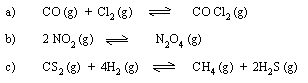(3) State Le-Chateliers principle (4) Describe the factors effecting chemical equilibrium using suitable examples. (5) State in which direction the equilibrium will be shifted by increasing the temperature as well as pressure on following equilibria.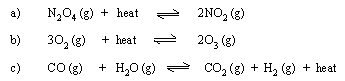(6) What temperature and pressure conditions would you recommend for the maximum yield of products in following reactions ? Give the reasoning for your recommendations.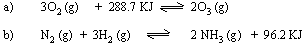(7) PCl5 (g) is 30.8% dissociated at 453 K and 202.6 KPa. Calculate the equilibrium constant using partial pressures. [ Ans. KP = 21.37 ] (8) 8 mol of Cl2 (g) and 7 mol of CO (g) are introduced into an empty 2 dm3 reaction vessel at a certain temperature.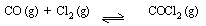At equilibrium there are 5.5 mol COCl2, calculate KC. [ Ans. 2.9 ] (9) The value of KC for the reaction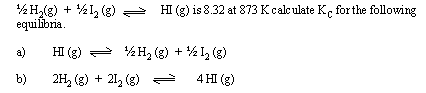[ Ans. a) 0.12 b) 4.791 ´ 103 ] (10) At 270C and 101.3 KPa N2O4 is 20% dissociated into NO2 , Find a) KP b) the % dissociation at 270C and total pressure of 10.13 KPa. [ Ans. a) Kp = 17.22 b) a = 55% ] Chapter 12 : Acids, Bases and Salts (1) Give different definitions of acid and base. (2) What are conjugate acids and bases. Give suitable examples. (3) Explain amphoteric nature of water. (4) Distinguish between i) Strong acid and weak acid ii) Strong base and weak base iii) Acid and base (5) What is pH of solution ? Explain pH scale. (6) Aqueous solution of sodium carbonate is basic and that of ammonium chloride is acidic. Explain with reactions. (7) Give scientific reasons : Dissociation of H2S is low in acidic media. (8) Give the formulae of any five strong acids, three strong bases and two each of weak acids and bases. (9) Classify the aqueous solution of the following substances as acidic, basic or neutral. CuSO4, FeCl3, KBr, NaI, Ch3COONH4, Na3PO4, K2SO4, HCN, NaCl, Al2(SO4)3, CH3COONa. (10) Arrange the following in decreasing order of pH 1 NHCl, 0.1 N HCl, H2O, 0.1 N H2SO4 (11) Calculate the hydrogen ion concentration of solution having pH = 6.495. [Ans. 3.199 ´ 10-7m ] (12) Find the pH of 0.2 m solution of H2SO4 assuming complete dissociation. [Ans. 0.398 ] (13) Calculate [OH-] of NH4OH solution having pH 9.75 at 298 K. [ Ans. 5.623´ 10-5 m ] (14) 0.01 m solution of acetic acid is 4.3% ionized at 198 K. Calculate pH of the solution. [ Ans. 3.37 ] (15) The dissociation constant of formic acid is 1.8 ´ 10-4. What is the degree of ionization in 0.01 m solution of this acid ? [ Ans. 0.1342 ] (16) The hydroxide ion concentration of a solution is 2.303 ´ 10-6 mol dm-3. Find the pH and indicate the nature of solution. [Ans. 8.362/ alkaline] (17) Find the pH of N/10 NH4OH. The dissociation constant is 1.8 ´10-5. [ Ans. 11.127 ] (18) Calculate the degree of dissociation of acetic acid is 1.342 ´ 10-2. [Ans. 1.8 ´ 10-5 m ] (19) Calculate [H+], [H2PO4 ], [HPO42], [PO43] and [H3PO4] in a 0.1 m solution of phosphoric acid. [ Ans. i) 2.4 ´ 10-2 m ii) 2.4 ´ 10-2 m iii) 6.2 ´ 10-2 m iv) 3 ´ 10-18 m v) 7.6 ´ 10-2 m ] (20) What are the concentrations of H+ , HC2O4- , C2O42- and H2C2O4 in 0.1 m solution of oxalic acid. (21) Give properties of acids and bases (22) Explain pH indicators (23) Give major uses of pH values. (24) Give different properties of the salt (25) Classify different salts with examples. Chapter 13 : Organic Chemistry (1) Show all the isomers of hexane as condensed structural formulas. (2) Classify organic compounds with suitable examples. (3) What ar carbocyctic and hetrocyclic compounds. Give two examples of each type. (4) What is functional group ? Name following function groups (i) -OH (ii) - C º N (iii) - COOH (iv) - SO3H (v) - CHO (vi) - CO - (5) Give general structures of the following compounds and functional group present in each of them. (i) Esters (ii) Amines (iii) Ethers (iv) Alkenes (v) Amides (vi) Acid chlorides. (6) What is homologous series ? (7) Differentiate between molecular formula and structural formula with examples. (8) Write structures of following compounds (i) Isobutane (ii) Neopentane (iii) cis - 2 - butene (iv) Methyl acetylene (v) Benzaldehyde (vi) Benzoyl chloride (9) Give structures of the following compounds and indicate which of them show geometrical isomerism. Draw structures of isomers if any. (i) 2 - Pentene (ii) 3 - Ethyl - 2- methyl - 3 - hexene (10) What is cis - trans isomerism ? Explain with suitable examples. (11) What are aromatic compounds. (12) Discuss the structure of benzene. ********** Index Chapter 1 Follow @Pinkmonkey_comYour browser does not support the IFRAME tag.
 Search: All Products Books Popular Music Classical Music Video DVD Toys & Games Electronics Software Tools & Hardware Outdoor Living Kitchen & Housewares Camera & Photo Cell Phones Keywords: